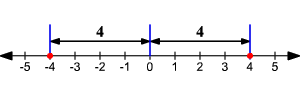# Absolute Value

The absolute value of a number is its distance from zero on a number line . For instance, $4$ and $-4$ have the same absolute value ( $4$ ).So, the absolute value of a positive number is just the number itself, and the absolute value of a negative number is its opposite. The absolute value of $0$ is $0$ .

Easy!

The absolute value of $x$ is written as $|\text{\hspace{0.17em}}x\text{\hspace{0.17em}}|$ . So,

$\begin{array}{l}|\text{\hspace{0.17em}}4\text{\hspace{0.17em}}|=4\\ |\text{\hspace{0.17em}}-4\text{\hspace{0.17em}}|=4\\ |54221.997|=54221.997\end{array}$

$|\text{\hspace{0.17em}}-\frac{1}{4}|=\frac{1}{4}$